# Species distribution mapping via data fusion

### Chapter 6: Case studies using hierarchical modelling

Data for this study are collected for $$n=741$$ grid cells covering the southeastern US. In each grid cell there are two observations of the presence of the brown-headed nuthatch (BHNU). For cell $$i$$, $$Y_{1i}\in\{0,1,…,N_{1i}\}$$ is the number of the $$N_{1i}$$ Breeding Bird Surveys (BBS) in cell $$i$$ for which a BHNU was observed; $$Y_{2i}\in\{0,1,2,…\}$$ is the number of sightings in $$N_{2i}$$ hours of eBird monitoring.

The true abundance (expected number of observed birds in one sampling occasion) in cell $$i$$ is denoted $$\lambda_i\ge 0$$. Abundance is related to the BBS data via the probability that a species is present and observed in cell $$i$$ on a given survey, $$p_i = 1-\exp(-\lambda_i)$$. To account for potential bias in the eBird we assume that the mean rate is $E(Y_{i2})/N_{i2}={\tilde \lambda}_i=\theta_1\lambda_i+\theta_2,$ where $$\theta_1$$ resolves the difference between BBS and eBird data and $$\theta_2$$ is the false positive rate. The data fusion model is $Y_{1i}|\lambda_i\sim\mbox{Binomial}(N_{1i},p_i) \mbox{ and } Y_{2i}|\lambda_i\sim\mbox{NegBinomial}(m,q_i)$ where $$q_i = \frac{m}{m+N_{i2}{\tilde \lambda}_i}$$. Since $$\lambda_i$$ appears in the likelihood for both data sources, both data sources are informative about the underlying abundance process.

The true intensity is modelled as $\log(\lambda_i) = \sum_{j=1}^pX_{ij}\beta_j$ where $$X_{ij}$$ are covariates and $$\beta_j\sim\mbox{Normal}(\beta_0 ,\sigma^2)$$ are the coefficients. In the absence of environmentally-relevant covariates, we select spline basis functions to model the spatial distribution of the BHNU. The basis functions are defined as outer products (one for longitude and one of latitude) of B-spline basis functions. To complete the Bayesian model we specify uninformative priors for remaining parameters. We let $$\beta_0\sim\mbox{Normal}(0,10)$$ and $$\sigma^2,\theta_1,\theta_2,m\sim\mbox{InvGamma}(0.1,0.1)$$.

load("S:\\Documents\\My Papers\\BayesBook\\Data\\Ebird\\BHNU.RData")
library(rjags)
library(fields)

set.seed(0820)

L     <- 10    # Number of basis functions
iter  <- 5000  # MCMC settings
burn  <- 1000
thin  <- 5

N1 <- BHNU$N_BBS_12 N2 <- BHNU$N_EBird_12
Y1 <- BHNU$Y_BBS_12 Y2 <- BHNU$Y_EBird_12
s  <- BHNU\$s
n  <- nrow(s)


## Plot the BBS data

BHNU_map(s,N1,main="BBS sampling occasions (N1)")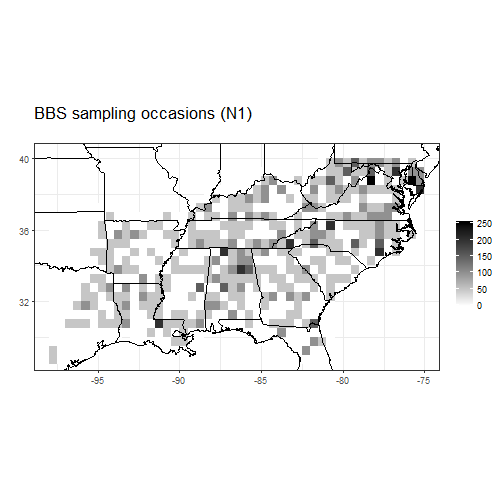BHNU_map(s,Y1,main="BBS counts (Y1)")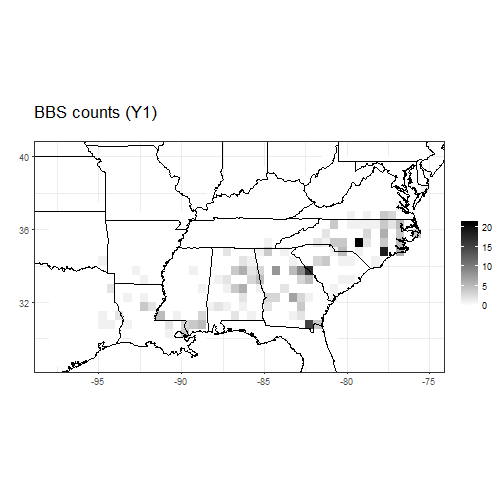BHNU_map(s,Y1/N1,main="BBS proportions (Y1/N1)")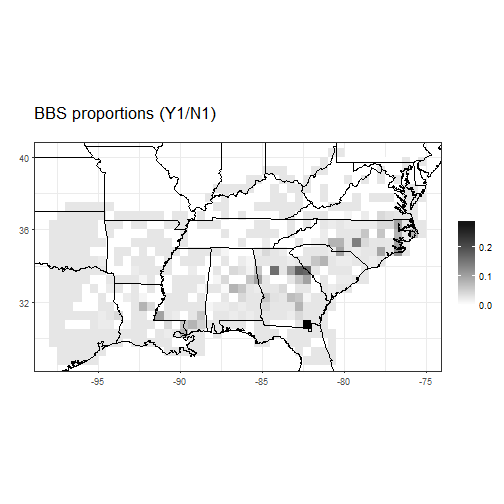Summary: Most of the sightings are in GA and SC, but the species distribution appears to extend as far north as VA and as far west as LA.

## Plot the eBird data

BHNU_map(s,N2,main="eBird effort (N2)")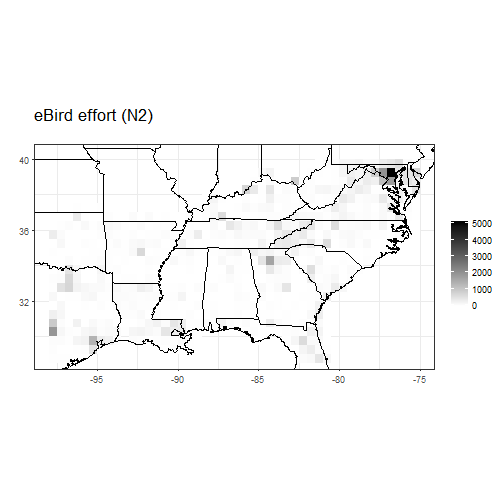BHNU_map(s,Y2,main="eBird counts (Y2)")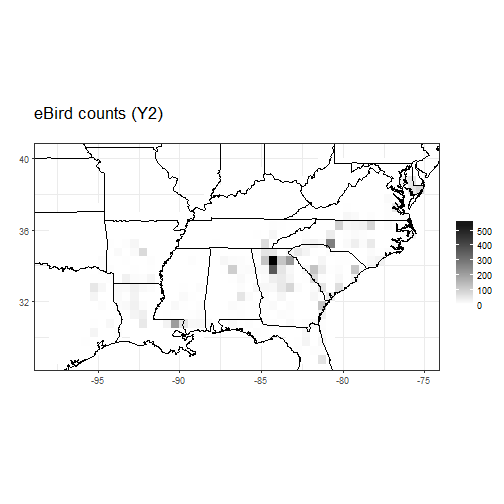BHNU_map(s,Y2/N2,main="eBird rates (Y2/N2)")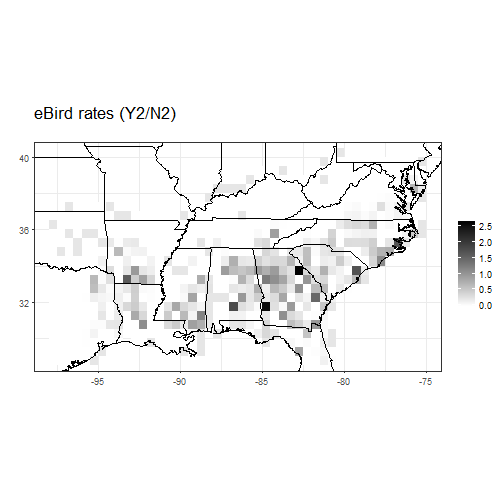Summary: The eBird maps generally agree with the BBS maps.

## Comparison of the data sources

plot(Y1/N1,Y2/N2,xlab="BBS proportion",ylab="eBird rate")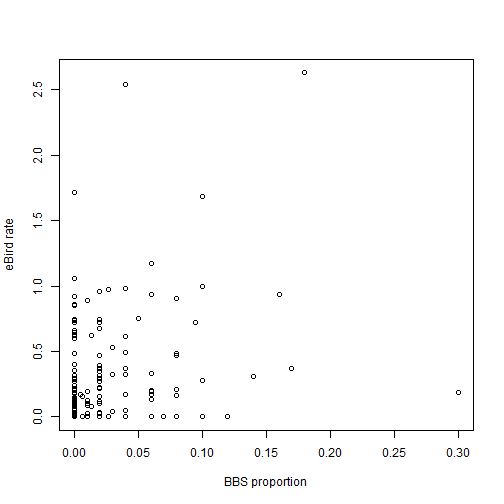Summary: There is some agreement between the two estimates, but a lot of noise. Spatial smoothing should help stabilize estimation.

## Set up the basis functions

library(splines)
B1   <- bs(s[,1],df=2*L,intercept=TRUE) # Longitude basis functions
B2   <- bs(s[,2],df=L,intercept=TRUE)   # Latitude basis functions
X    <- NULL
for(j in 1:ncol(B1)){for(k in 1:ncol(B2)){
X <- cbind(X,B1[,j]*B2[,k])  # Products
}}
X    <- X[,apply(X,2,max)>0.1]  # Remove basis function that are near zero for all sites
X    <- ifelse(X>0.001,X,0)
p    <- ncol(X)

BHNU_map(s,X[,10],main="A basis function")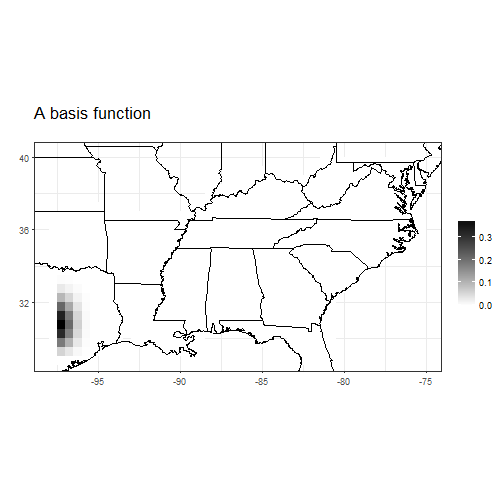BHNU_map(s,X[,20],main="A basis function")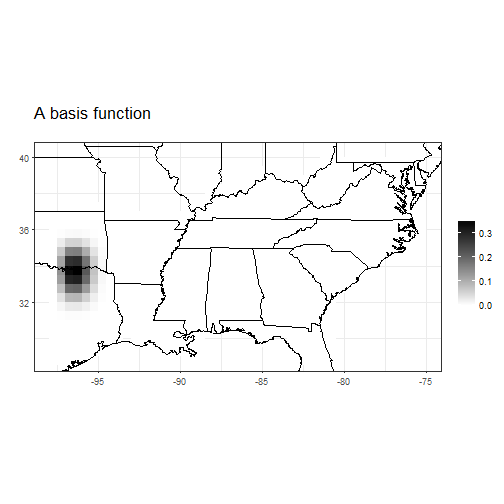BHNU_map(s,X[,21],main="A basis function")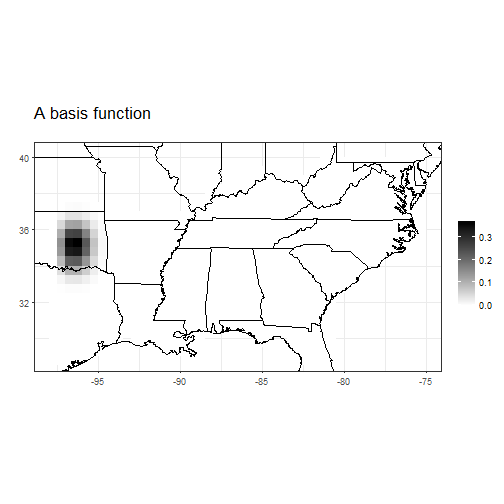## Put the data in JAGS format

id1  <- N1>0
id2  <- N2>0
data <- list(N1=N1[id1],Y1=Y1[id1],X1=X[id1,],
N2=N2[id2],Y2=Y2[id2],X2=X[id2,],
n1=sum(id1),n2=sum(id2),p=p)


## Specify the model

 model_string <- textConnection("model{

# BBS data
for(i in 1:n1){
Y1[i]             ~ dbin(phi1[i],N1[i])
cloglog(phi1[i]) <- max(-10,min(10,inprod(X1[i,],beta[])))
}

# eBrid data
for(i in 1:n2){
Y2[i]           ~ dnegbin(q[i],m)
q[i]           <- m/(m+N2[i]*(theta1*lam2[i]+theta2))
log(lam2[i])   <- max(-10,min(10,inprod(X2[i,],beta[])))
}

# Priors
for(j in 1:p){beta[j]~dnorm(beta0,tau)}
beta0   ~ dnorm(0,1)
tau     ~ dgamma(0.1,0.1)
theta1  ~ dgamma(0.1,0.1)
theta2  ~ dgamma(0.1,0.1)
m       ~ dgamma(0.1,0.1)
}")


## Fit the model in JAGS

 inits  <- list(theta1=10,theta2=1,beta0=-2,beta=rep(0,p),tau=100,m=1)
model  <- jags.model(model_string,data = data, inits=inits, quiet=TRUE, n.chains=2)
update(model, burn, progress.bar="none")
params <- c("beta0","beta","theta1","theta2","m","tau")
samps  <- coda.samples(model, variable.names=params,
n.iter=iter*thin, thin=thin, progress.bar="none")


## Convergence diagnostics

 sum    <- summary(samps)
ESS    <- effectiveSize(samps)
plot(ESS)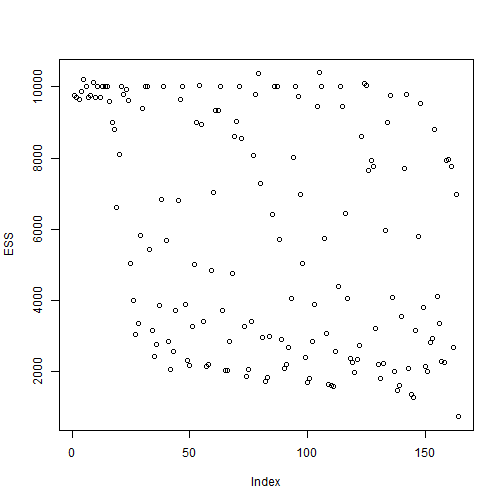## Plot estimated occupancy

 # Extract the samples of beta
beta   <- rbind(samps[][,1:p],samps[][,1:p])

# Reconstruct the draws of lambda
lambda <- beta%*%t(X)
lambda <- ifelse(lambda>10,10,lambda)
lambda <- exp(lambda)

lam_mn <- apply(lambda,2,mean)
occ    <- 1-exp(-lambda)
occ_p  <- colMeans(occ>0.01)

BHNU_map(s,lam_mn,main="Posterior mean abundance")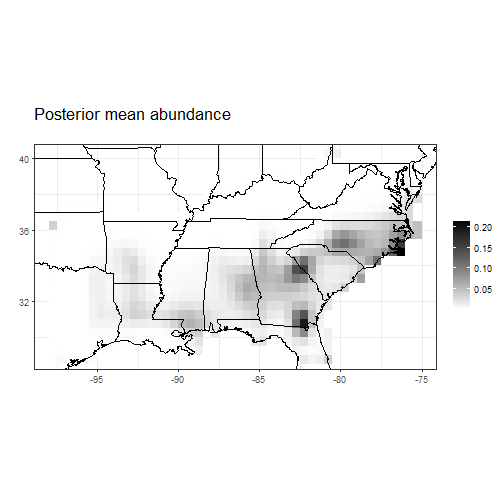BHNU_map(s,occ_p,main="Probability that occupancy exceeds 0.01")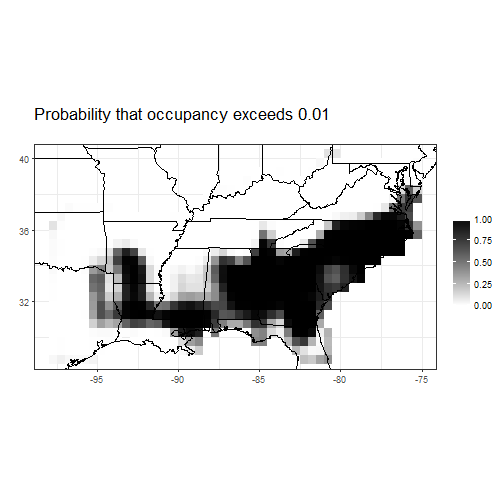Summary: As suggested by the maps of the raw data, the estimated BHNU distribution has highest probability in GA and the Carolinas, but high probability in the western part of the domain as well.